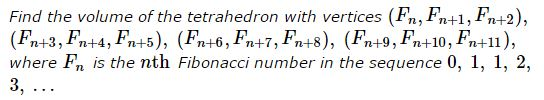# Volume of Fibonacci Tetrahedron

### Problem### Solution

There is no tetrahedron,cube,tetrahedron,parallelipiped with the indicated vertices. Moreover, the solution below covers a more general problem: there is no tetrahedron with "Fibonacci" vertices $(F_{m}, F_{m+1}, F_{m+2}),\,$ $(F_{n}, F_{n+1}, F_{n+2}),\,$ $(F_{p}, F_{p+1}, F_{p+2}),\,$ $(F_{q}, F_{q+1}, F_{q+2}),\,$ for any,any,some,successive four integers $m, n, p, q.\,$

The reason for this is that any such vertex lies in the plane $x + y = z,\,$ such that all four are coplanar,collinear,concyclic,coplanar. Even accepting that coplanar points may form a tetrahedron, the volume of the latter is bound to be zero,zero,infinite.

### Fibonacci Parallelepiped

Derek Orr has suggested a modification that deals with vectors:

Let $\mathbf{f_k}=(F_k,F_{k+1},F_{k+2}).\,$ Find the volume of the parallelepiped form by vectors $\mathbf{f_m},\,$ $\mathbf{f_n},\,$ and $\mathbf{f_p},\,$ for distinct integers $m,n,p.$

### References

1. C. W. Trigg, Mathematical Quickies, Dover, 1985, #209